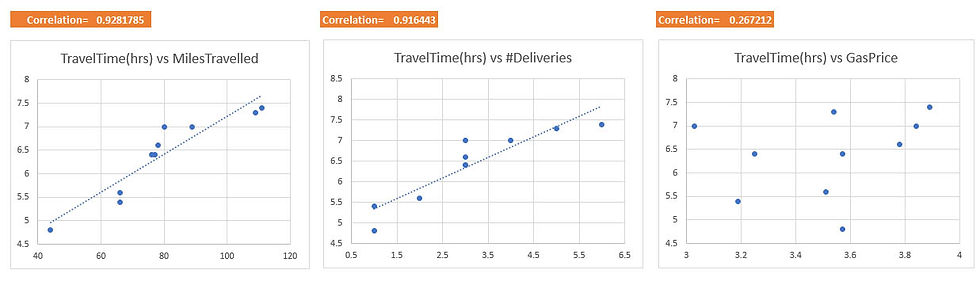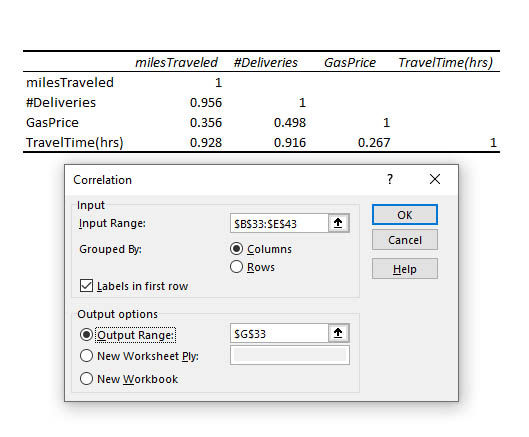top of page
Search
• Marek Vavrovic

# Multiple Linear Regression

Updated: Jan 3, 2021

Multiple linear regression model is the most popular type of linear regression analysis. It is used to show the relationship between one dependent variable and two or more independent variables.

In fact, everything you know about the simple linear regression modeling extends (with a slight modification) to the multiple linear regression models.

The following formula is a multiple linear regression model.

yi=β0​+β1​xi1​+β2​xi2​+...+βpxip​+ϵ

where, for i=n observations:

yi= dependent variable

xi= explanatory variables, independent variable

β0​= y-intercept (constant term)

βp= slope coefficients for each explanatory variable

ϵ= the model’s error term (also known as the residuals) ​

Example:

Let us assume that you are a small business owner for Regional Delivery Service (RDS) who offers same-day delivery for letters, packages, and other small cargo. You can use Google Maps to group individual deliveries into one trip to reduce time and fuel costs. Therefore, some trips will have more than one delivery.

As the owner, you would like to be able to estimate how long a delivery will take based on three factors:

1/ the total distance of the trip in miles

2/ the number of deliveries that must be made during the trip

3/ the daily price of petrol.Multiple regression is an extension of simple linear regression. Adding more independent variables to a multiple regression procedure does not mean the regression will be “better” or offer better predictions. In fact, it can make things worse. This is called overfitting.

The addition of more independent variables creates more relationships among them. So not only are the independent variables potentially related to the dependent variable, they are also potentially related to each other. When this happens, it is called multicollinearity. The ideal is for all the independent variables to be correlated with the dependent variable but not with each other.

Because of multicollinearity and overfitting, there is a fair amount of prep-work to do before conducting multiple regression analysis if one is to do it properly.

· Generate a list of potential variables: independent(s) and dependent

· Collect data on the variables

· Check the relationships between each independent variable and the dependent variable using scatterplots and correlations

· Check the relationships among the independent variables using scatterplots and correlations

· (optional) Conduct simple linear regression for each independent vs dependent pair

· Use the non-redundant independent variables in the analysis to find the best fitting model

· Use the best fitting model to make predictions about the dependent variable

3 relationships to analyzeScatter plot summary

Dependent variable vs independent variables:

· TravelTime(y) appears highly correlated with milesTraveled(x1)

· TravelTime(y) appears highly correlated with #Deliveries(x2)

· TravelTime(y) does not appear highly correlated with gasPrice(x3)

Since gasPrice(x3) does not appear correlated with the dependent variable we would not use that variable in the multiple regression.

Scatter plots:We must check the relationship among our 3 independent variables.We have detected a linear relationship between 2 independent variables: #Deliveries(x2) and MilesTraveled. This might cause a problem of MULTICOLLINEARITYIndependent variables scatterplot summary

· #Deliveries(x2) appears highly correlated with MilesTraveled(x1). This is multicollinearity.

· MilesTraveled(x1) does not appear highly correlated with gasPrice(x3)

· gasPrice(x3) does not appear correlated with #Deliveries(x2)

Since #Deliveries is HIGHLY CORRELATED with MilesTraveled, we would not use both in the multiple regression, they are redundant.

Correlations:

· TravelTime vs MilesTraveled, 0.928 = strong linear correlation

· TravelTime vs #Deliveries, 0.919 = strong linear correlation

· TravelTime vs GasPrice, 0.267 = no linear correlation

· MilesTraveled vs #Deliveries, 0.956 strong linear correlation between independent variables = multicollinearity. · In the regression we can substitute one for other. We will use only one of them.Correlation summary

Correlation analysis confirms the conclusions reached by visual examination of the scatterplots. Redundant multicollinear variables: MilesTraveled and #Deliveries are both highly correlated with each other and therefore are redundant. Only one should used in the multiple regression analysis. GasPrice is not correlated with the dependent variable and should be excluded.

Variable regressions

In this step we will perform a simple regression for each independent variable individually.

1/ TravelTime(y) vs MilesTraveled(x1)

Multiple R = strong linear correlation

R Square = 86.15% of the variation in the dependent variable is accounted by the independent variable. It is an indicator of how strength the model is.

Adjusted R Square = That is the same thing as R square. It is just adjusted for the number of independent variables in the model, which in this case is one. It will always be lower than R square.

Standard Error = Average distance of the data points from the regression line. Data points are on average 0.34 hours away from the regression line. Is in the units of the dependent variable, in this case hours.

Anova table

If P-value is less than 0.05 the model is significant

MilesTraveled Coefficient = 0.04. An increase in 1 mile will increase delivery time by 0.04 hours.

Regression equation

Ŷ = 3.1856 + 0.0403(MilesTraveled)

84-mile trip estimate

ŷ = 3.1856 + 0.0403(84)

ŷ = 6.5708 hours (6:34)

This is just an estimate. It is going to have some errors around it. We can find the prediction interval using t distribution.ŷ = 6.5708 ± 2.31(Standard Error)

ŷ = 6.5708 ± 2.31(0.3423)

ŷ = 5.7764 to 7.3615 hours

ŷ = 5:47 to 7:22 (95% PI)2/ TimeTraveled vs #Deliveries

TravelTime(y) = 4.845 + 0.4983(#Deliveries)

An increase in 1 delivery will increase delivery time by 0.4983 hours.

4 delivery estimate

ŷ = 4.845 + 0.4983(4)

ŷ = = 6.838 hours (6:50)3/ TimeTraveled vs gasPrice

Higher F value than better model we have, in this case F value is low. P value is > 0.05 this model is not significant.

Multiple R = Gas price has no linear relationship to travel time.Model options summary

The first independent variable MilesTraveled is the best for the regression model because It has the highest F value, lowest Standard Error value and highest R Square value.